## Square Root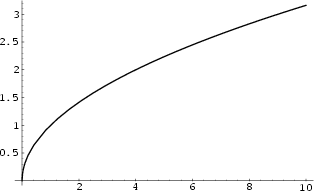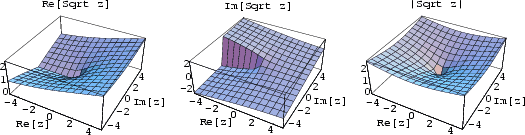A square root ofis a numbersuch that. This is written(to the 1/2 Power) or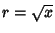. The square root functionis the Inverse Function of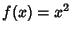. Square roots are also called Radicals or Surds. A general Complex Numberhas two square roots. For example, for the real Positive number, the two square roots are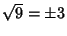, since. Similarly, for the real Negative number, the two square roots are, where i is the Imaginary Number defined by. In common usage, unless otherwise specified, the'' square root is generally taken to mean the Positive square root.

The square root of 2 is the Irrational Number(Sloane's A002193), which has the simple periodic Continued Fraction 1, 2, 2, 2, 2, 2, .... The square root of 3 is the Irrational Number(Sloane's A002194), which has the simple periodic Continued Fraction 1, 1, 2, 1, 2, 1, 2, .... In general, the Continued Fractions of the square roots of all Positive integers are periodic.

The square roots of a Complex Number are given by(1)

As can be seen in the above figure, the Imaginary Part of the complex square root function has a Branch Cut along the Negative real axis.

A Nested Radical of the formcan sometimes be simplified into a simple square root by equating(2)

Squaring gives(3)

so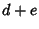(4)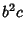(5)

Solving forand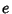gives(6)

A sequence of approximationstocan be derived by factoring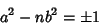(7)

(where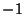is possible only ifis a Quadratic Residue of). Then(8)(9)

and(10)(11)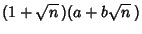(12)

Therefore,andare given by the Recurrence Relations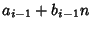(13)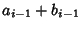(14)

with. The error obtained using this method is(15)

The first few approximants toare therefore given by(16)

This Algorithm is sometimes known as the Bhaskara-Brouckner Algorithm. For the case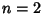, this gives the convergents toas 1, 3/2, 7/5, 17/12, 41/29, 99/70, ....

Another general technique for deriving this sequence, known as Newton's Iteration, is obtained by letting. Then, so the Sequence(17)

converges quadratically to the root. The first few approximants toare therefore given by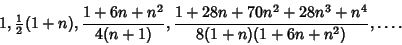(18)

For, this gives the convergents 1, 3/2, 17/12, 577/408, 665857/470832, ....

References

Sloane, N. J. A. Sequences A002193/M3195 and A002194/M4326 in An On-Line Version of the Encyclopedia of Integer Sequences.'' http://www.research.att.com/~njas/sequences/eisonline.html and Sloane, N. J. A. and Plouffe, S. The Encyclopedia of Integer Sequences. San Diego: Academic Press, 1995.

Spanier, J. and Oldham, K. B. The Square-Root Functionand Its Reciprocal,'' TheFunction and Its Reciprocal,'' and TheFunction.'' Chs. 12, 14, and 15 in An Atlas of Functions. Washington, DC: Hemisphere, pp. 91-99, 107-115, and 115-122, 1987.

Williams, H. C. A Numerical Investigation into the Length of the Period of the Continued Fraction Expansion of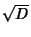.'' Math. Comp. 36, 593-601, 1981.